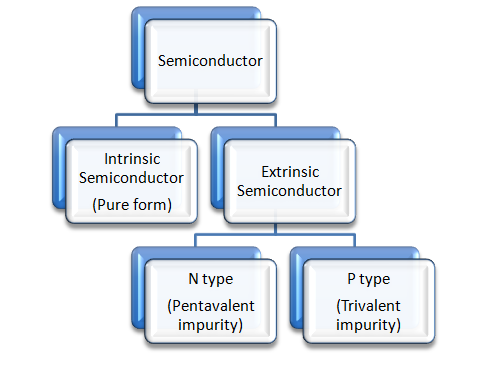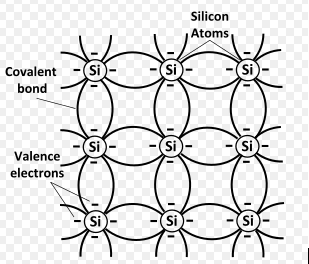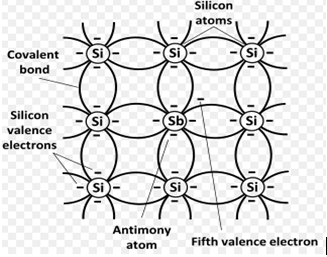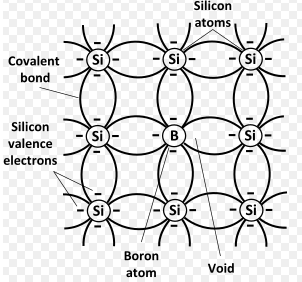### TYPES OF SEMICONDUCTOR:Types of Semiconductor

### INTRINSIC SEMICONDUCTOR:Intrinsic Semiconductor

Intrinsic semiconductor is a chemically pure semiconductor at room temperature . It is not doped or impurity is not added. Pure silicon(Si) and Germanium(Ge) are the example for intrinsic semiconductors. Its conductivity is very low at room temperature. The silicon and germanium have four valence electrons, to stabilize they share the valence electrons thus forming covalent bond. When the temperature is increased the covalent bond starts breaking and the valence electrons become free and start to move leaving a hole in the valence band. Thus the conductivity increases as the temperature is increased. The number of free electrons is equal to the number of holes formed in intrinsic semiconductor.

### EXTRINSIC SEMICONDUCTOR:

Extrinsic semiconductors are the semiconductors in which small amount of impurity is added and the impurity is called dopants. The process of adding impurity is called doping. By adding small amount of impurities its conductivity increases many times. The size of the dopants added are of similar size of the semiconductor atoms because it should not change the lattice structure of the semiconductor. The semiconductors are of fourth group elements. So the dopants added are from third group or fifth group elements. According to the impurity added there are two types of extrinsic semiconductors n type and p type.

#### n type extrinsic semiconductor:n type semiconductor

When the tetravalent (valence 4) elements  Si or Ge are doped with pentavalence (valence 5 ) like As , P elements  forms the n type extrinsic semiconductor. As we see in the figure when the pentavalent atoms takes the place of silicon, the valence 4 electrons forms the covalent bond with other 4 atoms and the 5th valence electron is free. This free electron is loosely bound and it moves freely even at the room temperature. When the temperature is increased even silicon atoms releases some of its valence electrons leaving holes. But always number of electrons are higher than the number of holes. Hence it is called as n type extrinsic semiconductor.

#### p type extrinsic semiconductor:p type semiconductor

When the trivalent elements like Al,B,Ga are doped with tetravalent elements like Si and Ge it forms p type semiconductor. As we see in the figure when Boron is doped with Si, the three valence electrons of Boron forms  a covalent bond with other three valence electrons of Si leaving a hole in the fourth valence electron. When the temperature is increased Si releases some of its valence electrons forming holes. But the number holes are more than the number of electrons, so holes are the majority charge carriers and hence they are called p type semiconductor.